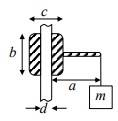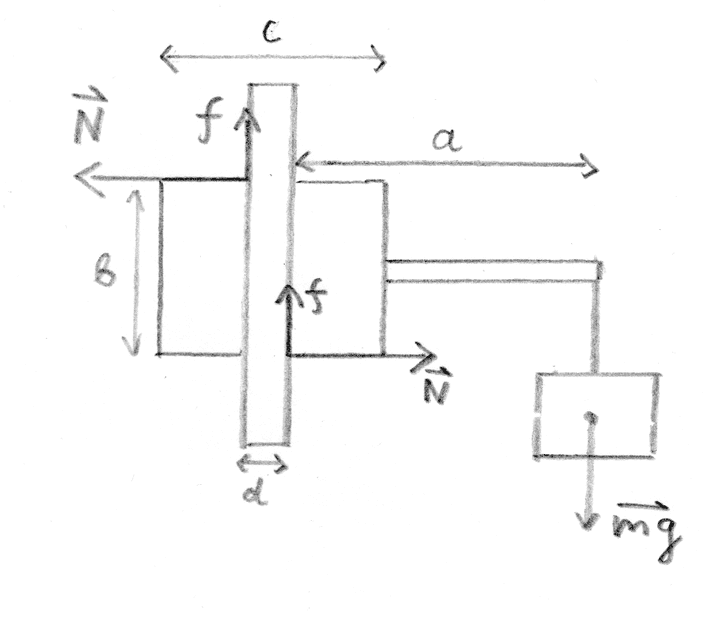# Moving nut on rod

• compaq65

#### compaq65

Homework Statement
There is a rod with a nut on it. Diameter of rod is d. There is a mass m object as shown in picture. all other parameters (a, b, c) are known. What coefficient of friction should be, so that nut is not moving.
Relevant Equations
Sum of moments and Newton I law.
I tried to draw a free-body diagram and sum of moments equal to zero, but I stuck there, because I don't know where should reaction force N be and how determine where friction is acting on round surface? Any ideas?Homework Statement:: There is a rod with a nut on it. Diameter of rod is d. There is a mass m object as shown in picture
When the problem says that there is a "nut" on the vertical rod, it seems that it is really talking about a "sleeve", "bushing" or "pair of plates on either side of the rod". This assembly is not tightly clamped onto the rod. Instead, it is free to slide up and down. However, it is machined or clamped to a tight tolerance so that it fits snugly and is not free to tilt or wobble as it slides. It presents zero frictional resistance unless a torque is present.

A "nut" would indicate the presence of threads. A "nut" would not be free to slide up and down on a threaded bolt.

We may begin by assuming that the assembly is in static equilibrium, just on the verge of slipping. Further, I would suggest assuming that the assembly itself has negligible mass -- gravity only acts on the attached mass m.
I tried to draw a free-body diagram and sum of moments equal to zero, but I stuck there, because I don't know where should reaction force N be and how determine where friction is acting on round surface? Any ideas?
You say that you have produced a free body diagram. May we see that diagram? Or can you at least list the moments that you have identified on that diagram and give us any equations that you have formed from them?

As it stands, you have showed no work -- only asserted that you have made some effort.

Edit: There is an additional assumption that may turn out to be necessary. Without it, the situation appears to be statically indeterminate: "Both contact patches are on the verge of slipping"

Last edited:
•BvU
When the problem says that there is a "nut" on the vertical rod, it seems that it is really talking about a "sleeve", "bushing" or "pair of plates on either side of the rod". This assembly is not tightly clamped onto the rod. Instead, it is free to slide up and down. However, it is machined or clamped to a tight tolerance so that it fits snugly and is not free to tilt or wobble as it slides. It presents zero frictional resistance unless a torque is present.

A "nut" would indicate the presence of threads. A "nut" would not be free to slide up and down on a threaded bolt.

We may begin by assuming that the assembly is in static equilibrium, just on the verge of slipping. Further, I would suggest assuming that the assembly itself has negligible mass -- gravity only acts on the attached mass m.

You say that you have produced a free body diagram. May we see that diagram? Or can you at least list the moments that you have identified on that diagram and give us any equations that you have formed from them?

As it stands, you have showed no work -- only asserted that you have made some effort.

Edit: There is an additional assumption that may turn out to be necessary. Without it, the situation appears to be statically indeterminate: "Both contact patches are on the verge of slipping"
I imagined that free-body diagram should look like this:So then we have

$$2f=mg$$
And if we choose left side upper point of a rod as rotation point

$$mg(a+d)=fd$$
Actually, I'm not quite sure how torque equations should look for this system.
And I cannot solve anything from the equations above.

I imagined that free-body diagram should look like this:
The diagram looks pretty good. Excellent, even. I had a different rotation axis in mind, but any choice will work.

[The axis I had in mind was in the middle of the nut, halfway between the two contact patches. The idea would be that this choice would let the ##f## drop out of the torque equation, hopefully simplifying things slightly. This would also make it obvious that you've missed accounting for the main torque].

You've properly identified the points of application of the relevant forces.
I agree with ##2f=mg## for the balance of linear forces.
I do not agree with ##mg(a+d) = fd## for the balance of torques. You have missed at least one torque.

You have not yet used the coefficient of friction or the assumption that both points of contact are on the verge of slipping.

You've assumed that both normal forces are identical and that both frictional forces are identical without making an argument that such must be the case. Not a big problem -- there is a simple argument that both must indeed be the case.

Last edited:
Well, if we suppose object is not moving, there are only to forces in y axis. So they must be equal. We supposing that coefficient of friction is equal everywhere, so that makes both friction forces equal.

If I make axis through a rod center. Equation becomes:
$$mg(a+\frac{d}{2})-N\frac{b}{2}-N\frac{b}{2}+f\frac{d}{2}-f\frac{d}{2}=0$$
I get final expression of friction coefficient as:
$$\mu =\frac{b}{2a+d}$$
Which is strange as it doesn't depend on mass.
Is the first equation right?

Last edited:
•jbriggs444
Well, if we suppose object is not moving, there are only to forces in y axis. So they must be equal. We supposing that coefficient of friction is equal everywhere, so that makes both friction forces equal.

If I make axis through a rod center. Equation becomes:
$$mg(a+\frac{d}{2})-N\frac{d}{2}-N\frac{d}{2}+f\frac{d}{2}-f\frac{d}{2}=0$$
I get final expression of friction coefficient as:
$$\mu =\frac{4d}{2a+d}$$
Which is strange as it doesn't depend on mass.
Is the first equation right?
I was all set to agree with your torque balance equation but it has a problem. What is the moment arm for the ##N##? It is not ##\frac{d}{2}##.

As for the coefficient of friction not depending on mass, that makes perfect sense. If you double the mass, you double all the forces. But coefficient of friction is not about forces. It is about force ratio. If you double all of the forces, their ratios all remain unchanged.

Also, from a dimensional analysis point of view, we are after a result that is a pure number. If the result actually changes when you change the only mass input in the problem then you'd expect to find mass units in the answer.

I was all set to agree with your torque balance equation but it has a problem. What is the moment arm for the ##N##? It is not ##\frac{d}{2}##.
Yes, my bad. It should be ##\frac{b}{2}##.

•jbriggs444
Thanks!

•jbriggs444
https://www.physicsforums.com/members/compaq65.731811/ said:
This user's profile is not available.
Was it something I said?

Was it something I said?
Nah, just a duplicate/banned account issue. Thanks for helping them; this thread is now closed.